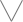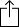C#
D#
E#
F#
G#
A#
B#
Chord
Scale
Custom
3nps
CAGED
None
Notes
Degrees
Intervals
None

1
2
3
4
5
1
2
3
4
5
6
7
8
9
10
11
12
13
14
15
16
17
18
19
20
21
22
23
24
E
B
G
D
A
E
B
F
E
F
G
G
A
A
B
C
C
D
D
E
E
F
G
G
A
A
B
C
C
D
D
E
C
C
D
D
E
E
F
G
G
A
A
B
C
C
D
D
E
E
F
G
G
A
A
B
G
A
A
B
C
C
D
D
E
E
F
G
G
A
A
B
C
C
D
D
E
E
F
G
D
E
E
F
G
G
A
A
B
C
C
D
D
E
E
F
G
G
A
A
B
C
C
D
A
B
C
C
D
D
E
E
F
G
G
A
A
B
C
C
D
D
E
E
F
G
G
A
E
F
G
G
A
A
B
C
C
D
D
E
E
F
G
G
A
A
B
C
C
D
D
E
C
C
D
D
E
E
F
G
G
A
A
B
C
C
D
D
E
E
F
G
G
A
A
B
G
G
A
A
B
C
C
D
D
E
E
F
G
G
A
A
B
C
C
D
D
E
E
F

C# Mixolydian Mode for 8 String Guitar - CAGED Chart

C# Mixolydian Mode is a diatonic scale and is the 5th mode in the set of Classical church modes

C
D
E
F
G
A
B
CW
W
H
W
W
H
W
Play scaleand then